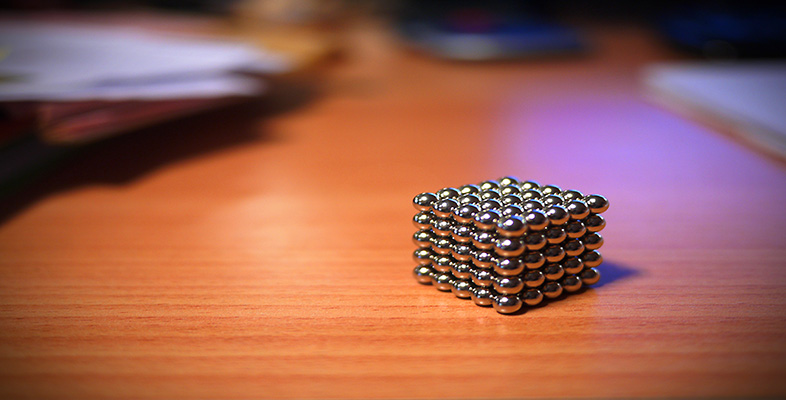Squares, roots and powers

Start this free course now. Just create an account and sign in. Enrol and complete the course for a free statement of participation or digital badge if available.

Free course

# 2.1.1 Try some yourself

## Activity 15

The size of a population of micro-organisms doubles every hour. If there are two of these creatures to start with, how many will there be after five hours?

After one hour there are 2 × 2 = 4 micro-organisms.

After two hours there are 2 × 4 = 8, or 2 × 2 × 2 = 23.After five hours there are 2 × 32 = 64, or 2 × 2 × 2 × 2 × 2 × 2 = 26.

So there will be 26 = 64 after five hours.

MU120_4M4